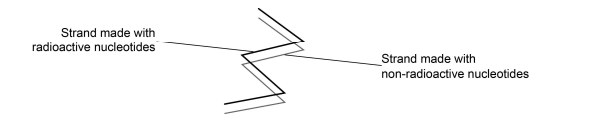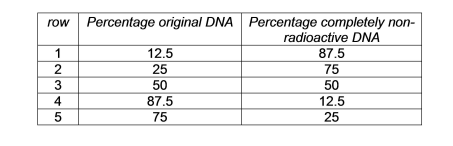# IMAT 2015 Q26 [Non-radioactive DNA]

A double stranded DNA molecule is shown below:This DNA molecule is allowed to replicate three times in a medium containing non-­radioactive nucleotides.

Which answer shows the correct percentages of original DNA and completely non-­radioactive DNA?A. row 4
B. row 1
C. row 5
D. row 3
E. row 2

The answer to this question is choice B.

Before we rationalize the answer, it would be useful to be reminded of semi-conservative DNA replication:

• Basically in this type of replication, an original DNA yields 2 copies, wherein each copy contains HALF of the original DNA. In short, if you have a double stranded DNA, if that undergoes replication, it will yield TWO double stranded copies, wherein each copy has a strand from the original DNA.

Let us then trace the strand of radioactive nucleotides as it replicates 3 times:

• Now that you are reminded of the concept of semi-conservative DNA replication, you will now be able to trace what happens when you replicate a strand that contains radioactive nucleotides. This will be made easier if we visualize this through the diagram below:

• To be oriented, the black lines = DNA strands made of NON-radioactive nucleotides, while the red lines = DNA strands made of RADIOACTIVE nucleotides.
• Do not forget, that the medium that was used was full of NON-radioactive nucleotides, which means that further replication will yield NEW strands that are made of non-radioactive nucleotides.
• After the 1st replication: This will yield 2 copies of DNA. As you would expect, there will be 2 DNA molecules, EACH having HALF of the original DNA. As you can see, 1 copy contains nothing but non-radioactive DNA, while the other copy contains the radiooactive half of the original DNA.
• After the 2nd and 3rd replication: Basically, after following the principle of semiconservative replication, you will have 8 total molecules of DNA, each copy of which contains half the DNA that they originated from.
• What is this question asking for? You will now need to calculate the proportion of DNA that contains RADIOACTIVE nucleotides! Let’s reread what the question is asking us to do: “correct percentages of original DNA and completely non-­radioactive DNA”. If you recall, the “original DNA” is the one that contains both a radioactive and non-radioactive strand. There are 8 total DNA copies after 3 replications. There are 7 completely non-radioactive DNA, while there is only 1 copy that is the same as the original DNA. A fraction of 1/8 would then give you a percentage of 12.5, which means that row 1 or choice B is the only correct answer.